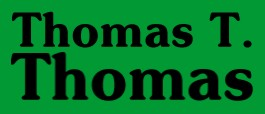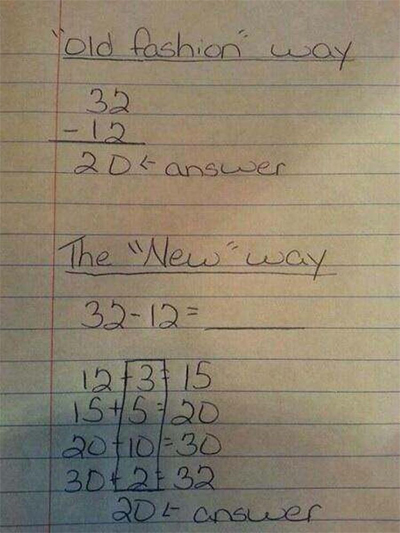Science and Religion

### Reduced Instruction Set Arithmetic – April 26, 2015I see recurring comments on Facebook and elsewhere about the new Common Core arithmetic, particularly the method of subtraction. People are indignant, confused, and upset, because this is a marked departure from the method we all learned in grade school.1

In the traditional method. you start by subtracting the numbers in the one’s column, and if the top number is smaller than the bottom number, you borrow a ten from the ten’s column, reducing the number there by an imaginary one. Proceed in like fashion across the pairs of top and bottom numbers in each decimal column, writing the result of each single subtraction below the line. Watch the result build up from the one’s column across out to however many places the answer happens to go.

This method reaches an exact answer most directly. So it’s good for accountants and other people who need to deal exclusively in exact quantities. But I was raised by an engineer. Now, I was never very good at math, no matter how hard my father tried, but one notion I did learn from him was the idea of approximation.2 That is, in most situations you don’t need the exact figure down to the one’s place and all the decimals,3 but instead you want a shot at the “close enough” answer without a lot of work. I think the Common Core teaching of subtraction is an approach to this.

In the Common Core method, you start with the lower number—the one you’re subtracting from the upper number—and add a comfortable, easily recognizable, rounded-off amount that takes you closer to the upper number. In the example most often given, subtracting 12 from 32, you add 3 to the starting 12 to get up to 15, then you add 5 to 15 to get to 20, then 10 to 20 to get to 30, then 2 to 30, to get to 32. Once you’ve made all these approximations, you add them up to get the answer: 3+5+10+2=20. And the difference between 32 and 12 is indeed 20.

This seems like a lot of work. And it is, if you go about it that way. A shorter route might be 3+12=15, 15+15=30, 2+30=32; so 3+15+2=20. Or 10+12=22, 10+22=32; so 10+10=20. In the Common Core, these would all be right answers—unless, of course, the hidden purpose of the exercise is to take the tiniest, most eensy-beansy steps in order to drive the child mad with all of those follow-up additions.

I wouldn’t teach children the Common Core method as the only way to subtract two numbers. First, because the “old way” is fast at reaching an exact answer. Second, because the child’s parents—at least for the next generation or so—will know the old way but not the new. Turning numbers on their heads is a dandy way to exclude parents from involvement with their children’s homework, or to blunt the child’s understanding when he or she hears a lot of parental muttering over an assignment.

But this method of approximating the answer from the bottom up is an excellent alternative to be taught alongside the old top-down subtraction. First, it teaches the child flexibility. It shows that the number system works both ways, and that subtraction can be thought of as another type of addition. Second, it works better with large numbers to get an approximate answer when a sense of proportion is all you’re actually looking for.

For example, suppose I have two large numbers: 53,246 and 34,152. I want to know approximately how much bigger the first is than the second. Say, I have two different makes and models of cars I’m thinking of buying, and I want to compare their two prices generally, without getting bogged down in the weeds with just a few dollars in pocket change. In the old way, I would start by subtracting 2 from 6 to get 4 and write down “4.” Then, because the upper number’s 4 in the ten’s column is smaller than the lower number’s 5, I would borrow 1 from the 2 in the hundred’s column, subtract 5 from 14 to get 9 and write down “9.” Then I would subtract 1 from 1 in the hundred’s column and write down “0.” All that work, and I’ve not yet reached the thousands—the biggest parts of both numbers.

With Common Core subtraction, I would say 34+20=54. That’s too large by a thousand, so I back that off to 34+19=53. The approximation is a difference of 19,000 and change. If I wanted an exact answer, I could go after those hundred’s, ten’s, and one’s places. And if the number there turned out to be negative, then I could subtract it from 19,000.

Accountants wouldn’t like this way of thinking. But a scientist managing data in terms of thousands and millions of cells, seedlings, or stars would find this a quick way to get into the right decimal ballpark. This is arithmetic you can do in your head, without paper and pencil and writing down phantom numbers loaned from one decimal place to another. It’s an instinctive way to get to the “big pieces” answer rather than starting out with the “little pieces.”

The Common Core method, because it turns subtraction into an addition problem, also strikes me as a kind of “reduced instruction set” arithmetic. Reduced instruction set is a concept from computer programming, where you trade a complex language full of purposefully designed operators for a simpler language with a limited number of operators. In this case, you’re trading an arithmetic with the operators “+” and “-” for a new arithmetic that only knows “+”. Generally, with reduced instruction set coding, as you trade off the complexity of discrete operators, you must accept the need to take more steps to achieve a result in any operation.

Say, for example, you want to program a robot car or forklift. The normal four instructions in a set for driving on a square grid—like a city’s streets or the aisles and rows of a warehouse—would be: Forward, Backward, Right Turn, Left Turn. The reduced instruction set would be would be: Forward and Right Turn. You could still perform all the necessary actions to navigate the regular blocks of a city like New York. But instead of backing up, you would take a turn around the block by executing Right, Right, Forward. And instead of making a left turn, you would execute Right, Right, Right, Forward.

Sounds stupid and silly, doesn’t it? Until you realize that UPS asks its drivers to do something similar in devising and following their delivery routes so that they can avoid hazardous, time-consuming left turns and U-turns in busy traffic.

The point of all this is not to be infuriating to parents or confusing to children, but to teach flexibility, to increase understanding, and ultimately to show that anyone can generally find more than one way to approach and solve a problem. And that’s always a useful teaching.

1. That is, all of us older than twelve.

2. I believe this comes—at least in my father’s generation—from their constant use of a slide rule. The old “slip stick” gives you rapid answers in multiplication and division, exponents, square roots, logarithms, trigonometric functions, and similar complex math. But it usually gives you just the “stripped down” version of the answer—that is, the most meaningful digits on the “big end” of the number, as in scientific and engineering notation. For example, the exact speed of light is 299,792,458 meters per second. But a scientist or engineer would write 3x108 or 3e8, or “about three million meters.” In working a slide rule for multiplication and division, you only get the significant figures and you generally have to keep track of the decimal places—the tens, thousands, millions, and billions—in your head.

3. Unless, of course, you are dealing with very tiny amounts to the right of the decimal point—in which case the significant figures are still written as a one-, two-, or three-place decimal but the exponent notation is negative, reflecting the number of zeroes between the actual answer’s decimal point and the leading significant figure.